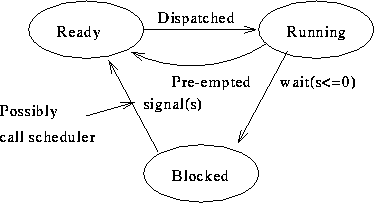# The Critical Section Problem; Solutions

Tom Kelliher, CS42

Sept. 30, 1996

# Software Solutions

## Two Process Solutions

### Try 1

Critical section code:

```int turn = 0;        /* shared control variable */

Pi:                  /* i is 0 or 1 */
while (turn != i)
;                 /* busy wait */
CSi;
turn = 1 - i;
```

1. Guarantees mutual exclusion.

2. Does not guarantee progress --- enforces strict alternation of processes entering CS's.

3. Bounded waiting violated --- suppose one process terminates while its its turn?

### Try 2

Remove strict alternation requirement

```int flag = { FALSE, FALSE }   /* flag[i] indicates that Pi is in its */
/*  critical section */

while (flag[1 - i])
;
flag[i] = TRUE;
CSi;
flag[i] = FALSE;
```

1. Mutual exclusion violated

2. Progress ok.

3. Bounded wait ok.

### Try 3

Restore mutual exclusion

```int flag = { FALSE, FALSE }   /* flag[i] indicates that Pi wants to */
/*  enter its critical section */

flag[i] = TRUE;
while (flag[1 - i])
;
CSi;
flag[i] = FALSE;
```

1. Guarantees mutual exclusion

2. Violates progress --- both processes could set flag and then deadlock on the while.

3. Bounded waiting violated

### Try 4

```int flag = { FALSE, FALSE }   /* flag[i] indicates that Pi wants to */
/*  enter its critical section */

flag[i] = TRUE;
while (flag[1 - i]) {
flag[i] = FALSE;
delay;                  /* sleep for some time */
flag[i] = TRUE;
}
CSi;
flag[i] = FALSE;
```

1. Mutual exclusion guaranteed

2. Progress violated (processes can ``dance'')

3. Bounded waiting violated

### Peterson's Algorithm

```int flag = { FALSE, FALSE }   /* flag[i] indicates that Pi wants to */
/*  enter its critical section */
int turn = 0;                    /* turn indicates which process has */
/*  priority in entering its critical */
/*  section

flag[i] = TRUE;
turn = 1 - i;
while (flag[1 - i] && turn == 1 - i)
;
CSi;
flag[i] = FALSE;
```

1. Satisfies all solution requirements

## Multiple Process Solution

Lamport's Bakery algorithm

```int choosing[NPROCS] = { FALSE }    /* cheating initializer */
int number[NPROCS] = { 0 }

choosing[i] = TRUE;
number[i] = max(number) + 1;
choosing[i] = FALSE;
for (j = 0; j < NPROCS; j++) {
while (choosing[j])
;
while (number[j] != 0 && (number[j] < number[i] ||
number[j] == number[i] && j < i) )
;
}
CSi;
number[i] = 0;
```

# Semaphores

1. Created by Dijkstra (Dutch)

2. A semaphore is an integer flag, indicating that it is safe to proceed.

3. Two operations:

1. Wait (p) --- proberen, test:
```   wait(s) {
while (s == 0)
;
s--;
}
```

Test and (possible) decrement executed atomically.

2. Signal (v) --- verhogen, increment:
```   signal(s) {
s++;
}
```

4. These are operations provided by the kernel. Wait and signal are atomic operations.

## Usage

1. Critical section solution:

```semaphore mutex = 1;

mutexbegin: wait(mutex);
mutexend:   signal(mutex);
```

1. By defn. of semaphore, mutual exclusion is achieved.

2. Also by defn. of semaphore, progress is achieved.

3. Bounded waiting depends on how the wait queue is implemented (if at all).

2. Interrupt signalling:

```semaphore sig = 0;

int_hndl:
signal(sig);

driver:
wait(sig);
```

3. Resource management (pool of buffers)

Producer/Consumer problem:

```semaphore count = N;
semaphore mutex = 1;

getbuf:
wait(count);               /* order important here */
wait(mutex);
<find empty buffer>
signal(mutex);
return(buffer);

relbuf:
wait(mutex);
signal(mutex);
signal(count);
```

4. Above semaphores inefficient --- spinlocks. Let waits which cause busy waits actually block the process:Associate a ``blocked'' queue with each semaphore.

```typedef struct semaphore {
int   value;
}
```

Semaphore creation:

```semaphore *createsem(int value) {

semaphore   *sem;

sem = get_next_sem();
sem->value = value;
return (sem);
}

void wait(semaphore *sem) {        /* need mutex goo here */

if (--sem->value < 0) {
<update status of current process>
scheduler();
}
}

void signal(semaphore *sem) {         /* mutex */

pcb   *proc;

if (++sem->value <= 0) {
<update status of proc>